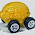## Tuesday, June 30, 2015

### The Euler equation as a maximum entropy condition

In the discussion of the RCK model on these two posts I realized the Euler equation could be written as a maximum entropy condition. It's actually a fairly trivial application of the entropy maximizing version of the asset pricing equation:

$$p_{i} = \frac{\alpha_{i}}{\alpha_{j}} \frac{\partial U/\partial c_{j}}{\partial U/\partial c_{i}} p_{j}$$

To get to the typical macroeconomic Euler equation, define $\alpha_{i}/\alpha_{j} \equiv \beta$ and re-arrange:

$$\frac{\partial U}{\partial c_{i}} = \beta \; \frac{p_{j}}{p_{i}} \; \frac{\partial U}{\partial c_{j}}$$

The price at time $t_{j}$ divided by the price at time $t_{i}$ is just (one plus) the interest rate $R$ (for the time $t_{j} - t_{i}$), so:

$$\frac{\partial U}{\partial c_{i}} = \beta (1 + R) \; \frac{\partial U}{\partial c_{j}}$$

And we're done.

The intuition behind the traditional economic Euler equation is (borrowed from these lecture notes [pdf])
The Euler equation essentially says that [an agent] must be indifferent between consuming one more unit today on the one hand and saving that unit and consuming in the future on the other [if utility is maximized].
The intuition for the maximum entropy version is different. It does involve the assumption of a large number of consumption periods (otherwise the intertemporal budget constraint wouldn't be saturated), but that isn't terribly important. The entropy maximum is actually given by (Eq. 4 at the link, re-arranged and using $p_{j}/p_{i} = 1 + R$):

$$c_{j} = c_{i} (1 + R)$$

The form of the utility function $U$ allows us to transform it into the equation above, but this is the more fundamental version from the information equilibrium standpoint. This equation says that since you could be anywhere along the blue line between $c_{j}$ maximized and $c_{i}$ maximized on this graph:

the typical location for an economic agent is in the middle of that blue line . Agents themselves might not be indifferent to their location on the blue line (or even the interior of the triangle), but a maximum entropy ensemble of agents is. Another way to put it is that the maximum entropy ensemble doesn't break the underlying symmetry of the system -- the interest rate does. If the interest rate was zero, all consumption periods would be the same and consumption would be equal. A finite interest rate transforms both the coordinate system and the location of maximum entropy point. You'd imagine deforming the n-dimensional simplex so that each axis was scaled by $(1 + r)$ where $r$ is the interest rate between $t_{i}$ and $t_{i + 1}$.

Footnotes:

 The graph shown is actually for a large finite dimensional system (a large, but finite number of consumption periods); the true entropy maximum would fall just inside the blue line/intertemporal budget constraint.

1.Although good of you to note, it leaves me feeling somewhat incomplete, yes we can take the interest rate as exogenous....how do you pick the interest rate?...usually we need the euler equation and the production function...

1.I could probably add in the interest rate from here:

http://informationtransfereconomics.blogspot.com/2015/05/dynamics-of-savings-rate-and-solow-is-lm.html

In any case, this was just a piece of a full model, not a model in itself :)

2.This comment has been removed by the author.